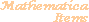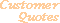Home Older Mathematica Items

Scientific Arts

Maxwellian Probability Distribution Function

Page 1 | Page 2 | Page 3 | Page 4

Interpolation Function Method

We need to invert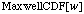. Here is a plot.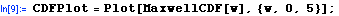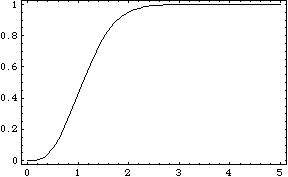We see that the cumulative is quite close to 1 by the time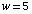. The error (the "left over probability") in stopping at this value of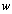is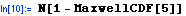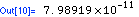If we sampleat a sequence of points, we can form ordered pairs of those points with the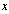and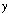order reversed. From a list of such points we can then form an InterpolatingFunction object through the use of Mathematica's Interpolation function. One way to do this is to just use the Table function as in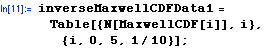This method samples MaxwellCDF at regularly separated points. Another way to do the sampling is to make use of the adaptive algorithm that is used internally by the Plot function. The Graphics object returned by the Plot function above (and abbreviated as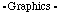in the output) contains within it the sampled data points that Plot's internal algorithm obtained. We can see the structure of CDFPlot by looking at its InputForm (and we wrap it in the function Short so that not all of its contents are displayed).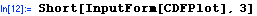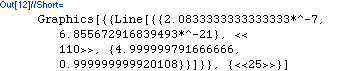The data that we are interested in is contained in the argument of the Line graphics primitive. This can be accessed by just taking the correct Part of CDFPlot. Once we have this we need to reverse theandorder: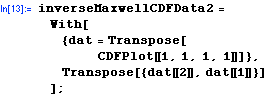In the above function we extracted the data from the Graphics function by directly accessing the needed Part of the expression. Another way to do this is to use pattern matching and transformation rules as follows.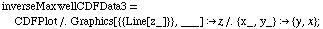then,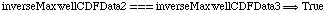.

We can now define an inverse function (using the data that was generated by the Plot command).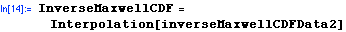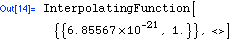This interpolation function is not well defined outside of the range of the sample points, given by the first argument of the InterpolatingFunction (the second argument is suppressed in the output).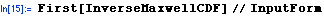{{6.855672916839493*^-21, 0.999999999920108}}

Here is an example of a Maxwellian random number.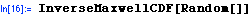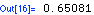So we can extend the Random function. We associate this extension with MaxwellPDF rather than Random.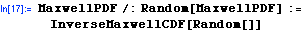Now let's generate a list of 5000 Maxwellian random numbers.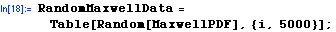To look at this data we can histogram it. First we load the standard add-on package, Statistics`DataManipulation`.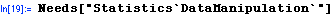Now we can use the function BinCounts.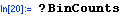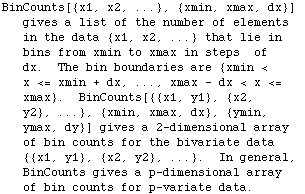Here is the binned data.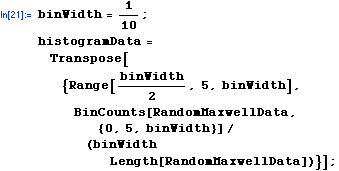Let's visualize the result in the form of a histogram along with a plot of MaxwellPDF for comparison.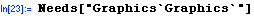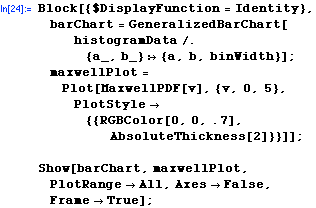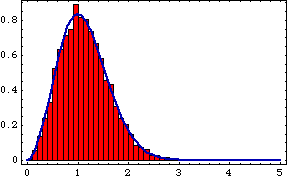<<PreviousNext >>

 For further information on our services send email to info@scientificarts.com . Contents of this web site Copyright © 1999-2011, Scientific Arts, LLC.
 s